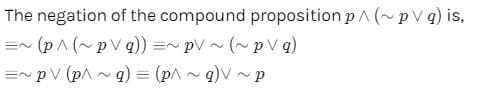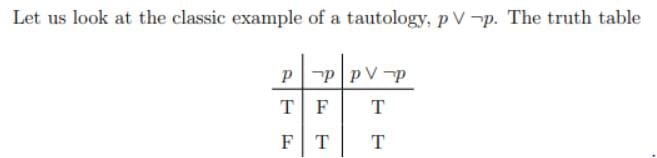Test: Mathematical Reasoning- 2

# Test: Mathematical Reasoning- 2

Test Description

## 25 Questions MCQ Test Mathematics (Maths) Class 11 | Test: Mathematical Reasoning- 2

Test: Mathematical Reasoning- 2 for Commerce 2023 is part of Mathematics (Maths) Class 11 preparation. The Test: Mathematical Reasoning- 2 questions and answers have been prepared according to the Commerce exam syllabus.The Test: Mathematical Reasoning- 2 MCQs are made for Commerce 2023 Exam. Find important definitions, questions, notes, meanings, examples, exercises, MCQs and online tests for Test: Mathematical Reasoning- 2 below.
Solutions of Test: Mathematical Reasoning- 2 questions in English are available as part of our Mathematics (Maths) Class 11 for Commerce & Test: Mathematical Reasoning- 2 solutions in Hindi for Mathematics (Maths) Class 11 course. Download more important topics, notes, lectures and mock test series for Commerce Exam by signing up for free. Attempt Test: Mathematical Reasoning- 2 | 25 questions in 25 minutes | Mock test for Commerce preparation | Free important questions MCQ to study Mathematics (Maths) Class 11 for Commerce Exam | Download free PDF with solutions
 1 Crore+ students have signed up on EduRev. Have you?
Test: Mathematical Reasoning- 2 - Question 1

### The contrapositive of (p∨q)→ r is

Detailed Solution for Test: Mathematical Reasoning- 2 - Question 1

Contrapositive of p→q is ∼q→∼p.
∴ Contrapositive of (p∨q)⇒r  is ∼r⇒∼(p∨q) i.e. ∼r⇒(∼p∧∼q).

Test: Mathematical Reasoning- 2 - Question 2

### The contrapositive of p→(∼q→∼r) is

Test: Mathematical Reasoning- 2 - Question 3

### Let p and q be two propositions. Then the inverse of the implication p→q is

Test: Mathematical Reasoning- 2 - Question 4

Let p and q be two propositions. Then the contrapositive of the implication p→q is

Test: Mathematical Reasoning- 2 - Question 5

Let p and q be two propositions. Then the implication ∼(p↔q)∼(p↔q) is :

Test: Mathematical Reasoning- 2 - Question 6

p∧(q∧r) is logically equivalent to

Test: Mathematical Reasoning- 2 - Question 7

Which of the following proposition is a tautology ?

Test: Mathematical Reasoning- 2 - Question 8

The negation of the compound statement p∨(∼p∨q) is

Detailed Solution for Test: Mathematical Reasoning- 2 - Question 8Test: Mathematical Reasoning- 2 - Question 9

The proposition p→∼(p∧∼q) is

Test: Mathematical Reasoning- 2 - Question 10

The proposition (p→∼p)∧(∼p→p) is

Test: Mathematical Reasoning- 2 - Question 11

Which of the following is equivalent to (p∧q) ?

Test: Mathematical Reasoning- 2 - Question 12

Which of the following is logically equivalent to ∼(∼p→q) ?

Detailed Solution for Test: Mathematical Reasoning- 2 - Question 12

∼(p⇒q)≡p∧∼q
∴ ∼(∼p⇒q) ≡∼p∧∼q

Test: Mathematical Reasoning- 2 - Question 13

The contrapositive of the inverse of p⇒ ~q is

Detailed Solution for Test: Mathematical Reasoning- 2 - Question 13

The inverse of p ⇒ ∼q is ∼p ⇒ q
The contrapositive of ∼p ⇒ q is ∼q ⇒ p. [∴ Contrapositive of p ⇒ q is∼q ⇒ p.]

Test: Mathematical Reasoning- 2 - Question 14

p→q is logically equivalent to

Test: Mathematical Reasoning- 2 - Question 15

The contrapositive of (∼p∧q)→ is

Test: Mathematical Reasoning- 2 - Question 16

The negation of p∧∼(q∧r) is

Test: Mathematical Reasoning- 2 - Question 17

The negation of q∨∼(p∧r) is

Test: Mathematical Reasoning- 2 - Question 18

Which of the following is a contradiction?

Test: Mathematical Reasoning- 2 - Question 19

Which of the following is logically equivalent to (p∧q) ?

Test: Mathematical Reasoning- 2 - Question 20

The negation of the proposition q∨∼(p∧r) is

Test: Mathematical Reasoning- 2 - Question 21

If p→(q∨r) is false , then the truth values of p , q and r, are respectively

Test: Mathematical Reasoning- 2 - Question 22

The statement p ⇒ p∨q is

Detailed Solution for Test: Mathematical Reasoning- 2 - Question 22

A tautology is a proposition is always true.Test: Mathematical Reasoning- 2 - Question 23

The statement p∨qp∨q is

Test: Mathematical Reasoning- 2 - Question 24

Which of the following sentences is a statement ?

Test: Mathematical Reasoning- 2 - Question 25

Let p and q be two prepositions given by p : I take only bread and butter in breakfast. q : I do not take anything in breakfast. Then , the compound proposition “ I take only bread and butter in breakfast or I do not take anything “ is represented by

## Mathematics (Maths) Class 11

156 videos|176 docs|132 tests
Information about Test: Mathematical Reasoning- 2 Page
In this test you can find the Exam questions for Test: Mathematical Reasoning- 2 solved & explained in the simplest way possible. Besides giving Questions and answers for Test: Mathematical Reasoning- 2, EduRev gives you an ample number of Online tests for practice

## Mathematics (Maths) Class 11

156 videos|176 docs|132 tests(Scan QR code)